# How to Calculate Years, Months, Days elapsed from a certain date in Microsoft Excel

In this article, we will learn how to calculate the number of years, months and days elapsed from a certain date using DATEDIF function in Excel.

We will learn how to use Today function to get Today’s date in Excel.

DATEDIF function returns the number of years, months and days between the given two dates in Excel.

Syntax:

=DATEDIF(start_date, end_date, unit)

Unit:

“y” denotes Years between dates
“m” denotes between dates
“d” denotes days between dates
"md" denotes days between dates, ignoring months and years
"ym" denotes months between dates, ignoring days and years
"yd" denotes days between dates, ignoring years

TODAY function in Excel is used to generate today’s date in a cell.
Syntax:

=Today()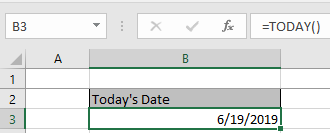Let’s get this with an example shown below.
Here we need to calculate the age of Ned Stark’s children.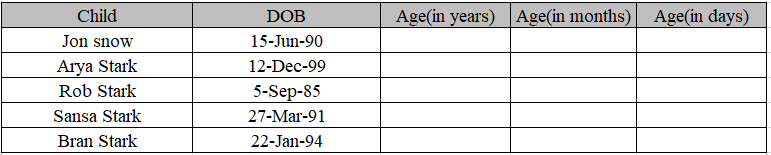Use the formula to get the age of children.
Formula:

=DATEDIF(C4,TODAY(),"y")

Date of birth is the start date in the C4 cell.
Today() function is used to get Today’s date as an end date.
“Y” to get the age in years.

Note:
Here we are using Today as an end date. You can use end date as your choice.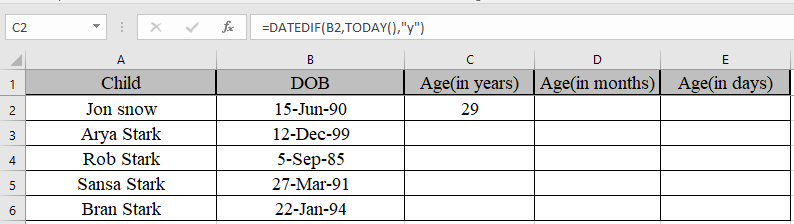We got the age of Jon Snow using DATEDIF function
Copy the formula in other cells, select the cells taking the first cell where the formula is already applied, use shortcut key Ctrl + D to find the age of rest of the children.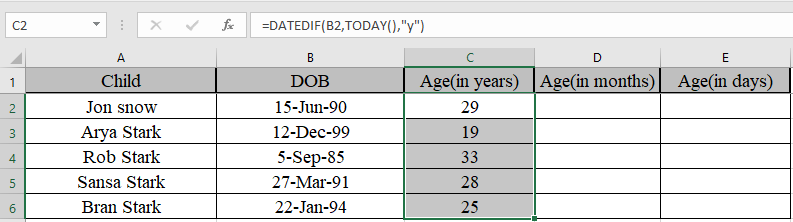Similarly, we will find out months and days using the same function but different unit.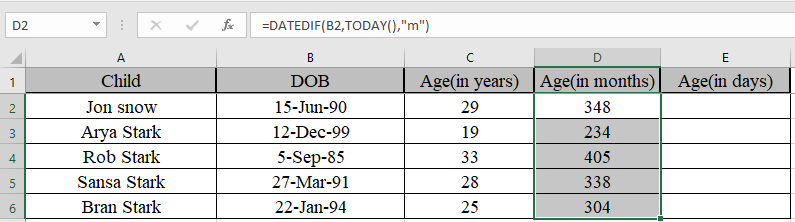We got the age in months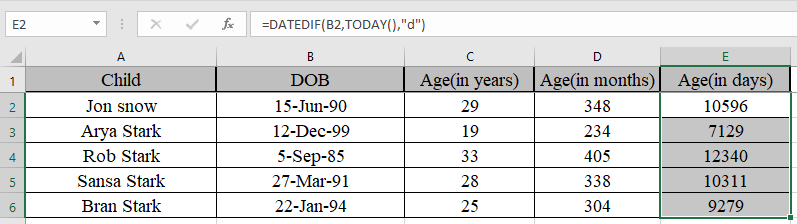We got the age in days

Now Some of you are wondering if we need to find the years, months and days between the two dates.

Use the formula to get the years, months and days together

=DATEDIF(B2,TODAY(),"y") & "y " &
DATEDIF(B2,TODAY(),"ym")&"m " &
DATEDIF(B2,TODAY(),"md") & "d"

Explanation:
DATEDIF(B2,TODAY(),"y") & "y " denotes years between dates with string “y ” attached to the number
DATEDIF(B2,TODAY(),"ym")&"m " denotes months between dates, ignoring days and years with string “m ” attached to the number.
DATEDIF(B2,TODAY(),"md") & "d" denotes days between dates, ignoring months and years with string “d” attached to the number.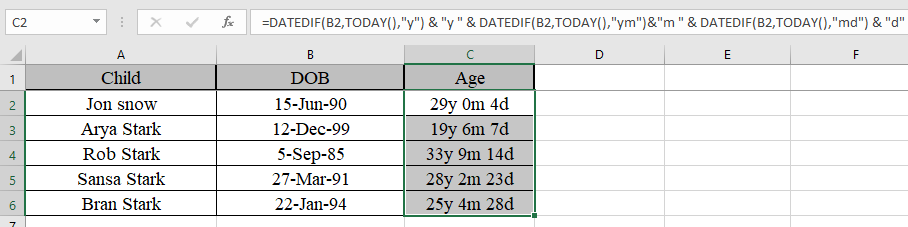As you can see their age now. You can use this function to calculate the number of days in service in Excel.

Hope you understood how to calculate the number of years, months and dates from a certain date. You can perform these tasks in Excel 2013 and 2010 workbook. Please share your query below in the comment box. We will assist you.

Popular Articles:

50 Excel Shortcuts to Increase Your Productivity

How to use the VLOOKUP Function in Excel

How to use the COUNTIF function in Excel

How to use the SUMIF Function in Excel

1.Hello

I have three columns. Birthday, Member since, and TODAY's date.
I want to calculate how many birthdays this person has had with us since he is member of our program.
Anyone can help me?

2.Do you know a formula that grants an upload of PTO at the 1st of every year? In other words if an employee has been employed for 1 year then they will receive a lump sum of days at the beginning of calendar year. Days granted will change according to their tenure.

3.Look-out!

Formula "=INT((TODAY()-A3)/365.25) & ” years , ” & INT(MOD((TODAY()-A4)/365.25,1)*12) & ” months and ” & INT(MOD((TODAY()-A4)/30.4375,1)*30.4375) & ” days” " is not the same like formula:
=DATEDIF(S104,(TODAY()),”y”) & ” years, ” & DATEDIF(S104,(TODAY()),”ym”) & ” months, ” & DATEDIF(S104,(TODAY()),”md”) & ” days”

especially in leap-years.
See how it works on dates:
2016-02-01 and 2016-03-02
and
2017-02-01 and 2017-03-02

4.Hi,

Can any help on this:

If we have 2 columns : previous experience and current exp. than how can we add the values.

Example: My previous experience is 2.11 Years and my current Experience is 3.10 Years

5.Raghavan Shridhar

If i have a number of days available and if i have to know the date of joining of the employee how do i calculate in reverse please help

6.Birth Date: January 15, 1988
Age at the date of: December 7, 2015
Age: 27 years. 10 months and 22 days

7.Oh YEAH! Well...

Birth Date: June 10, 2000
Age at the date of: December 7, 2015
Age: 15 years, 5 months and 27 days

8.Hi!
Thank you for the article! In a range of dates I need to know how many days there are in one month and how many days in the following month!
Example:
30/05/2015-13/06/2015 : 2 days in May and 12 days in June.
Is it possible? Thank you 😉

9.Hi, I'm trying to perform what should be quite a simple function in Excel.
I have 3 columns -
Invoice Date : Payment Terms (Days) : Payment Due
The values of these columns are -
05/01/15 : 30 : ???
How do i work out what the payment due date is in excel via a formula?

Would love some help here - struggling to create the right formulas for this one - I'm quite a basic user and this uses some differing numerical formats.

Many thanks!

•Hi,

Thanks

10.Try this formula, it actually works. S104 is the date you are counting from, you can replace the (TODAY()) Reference with another cell containing a date. As mentioned there are issues with the DATEDIF formula, missing days etc, but is accurate for most uses.

=DATEDIF(S104,(TODAY()),"y") & " years, " & DATEDIF(S104,(TODAY()),"ym") & " months, " & DATEDIF(S104,(TODAY()),"md") & " days"

•11.So, I keep trying to use this formula to give me "years, months, and days"; it is giving me nothing but errors (#NAME?) . I even tried to emulate your exact numbers and not working. Is there something I'm missing? Did the formula on here have extra spaces? This is a feature I would absolutely love to be able to use for my company.

•S Chandrasekar

It seems you are using a negative number in the answer cell. Please ensure that the starting date is smaller than or equal to the ending date.

12.I am trying to put in a conditional format that shows red when 3 years is up on a date already in the spreadsheet. Can you help?

13.I'm trying to calculate three date ranges so I used the following formula but it is off by 1.5 years:

=INT(((B4-A4)+(B5-A5)+(B6-A6))/365.25) & " years, "& INT(MOD((B4-A4)+(B5-A5)+(B6-A6)/365.25,1)*12) & " months and "&INT(MOD((B4-A4)+(B5-A5)+(B6-A6)/30.4375,1)*30.4375) &" days"

Any suggestion?

14.How can i do if i want: input Date In Cell A1 and then in Cell A2 increase month every month .
Ex: Cell A1 : 25-7-2014
and Cell A2 : 25-8-2014 and one month ago
Cell A2 : 25-8-2014
Thank if can please sent to my email
Keomoyura2011@Gmail.com

15.sir i have an problem with my work.i want to send my work by automatically. if we do not done today work so how can i send it to next day.

16.Tammy Garrison

I work in a school setting in special education and each child must have a new evaluation every three years.

I need a formula to count down the remaining time between evaluations and that would show the evaluations already done from a given date as a negative number or different color or something. Results need to be in a year/month/day or year.month.day format.

17.I work in human resources and I am trying to keep track of how many years my employees have been with us at any given time. I have not been able to figure out how to get the elapsed years between the two dates. I have manually done the DATEDIF function, and yes it gives me the desired answer but if I change the current date, the amount of years does not automatically update. I am getting really frustrated so please help. Also if you can reply by email that would be wonderful. Thank you so much.

•=INT((TODAY()-A1)/365.25) & " Yrs , " & INT(MOD((TODAY()-A1)/365.25,1)*12) & " Mths"

N PUT THIS FORMULA IN A2..IF YOUR JOINED DATE IS IN A1 (SO EVERYTIME THE JOINED DATE CHANGE THE ANSWER IN CELL A2 CHANGE.

18.Dear Sir Pls Tell me that how to find battery life in Excel format......?

Exam....
Date of Sale is - 28/05/2011
Date od Complaint is - 03/05/2014
-------------------------------------
Total used Warranty = ? / ? / ?
-------------------------------------

19.How can calculate year &month in continus next row. ie.

dec.13 =30 years
jan.14 =30 years 1 Month
feb.14 =30 years 2 month
asnd so on upto the age of 65 years.